• 学习曲线Learning Curve、常见学习曲线形态及解读、验证曲线Validation Curve、验证曲线+学习曲线对比
2021-05-31 14:24:06

# 学习曲线Learning Curve、常见学习曲线形态及解读、验证曲线Validation Curve、验证曲线+学习曲线对比

目录机器学习 深度学习 数据挖掘 神经网络 算法
更多相关内容
• 机器学习之模型调优的评估工具 验证曲线 学习曲线

# 学习目标：

在学习网格搜索，交叉验证之后，对模型优化的效果进行图形化的评价的两种工具：

• 验证曲线： 由于训练集的评分已经被用于参数调优，因此该评分用于评估效果已经不再客观，需要使用验证集的评分用于评估。
sklearn.model_ selection.validation_ curve(
estimator, X, y
param_ name : string, 在模型搜索中需要改变的参数
param_ range : array-like, shape (n_ values,), 相应参数的具体值列表

groups = None : array-like, with shape (n_ samples, )
拆分为训练/测试集时用到的样本分组标签
cv = None : int, 交互验证时的分组方法，为None时按照三组拆分

scoring = None, n_ jobs = 1，pre_ dispatch = ‘all’, verbose = 0
)返回:
train_ scores : array, shape (n_ ticks, n_ cv folds)， 测试集的模型评分
test_ scores : array, shape (n_ ticks, n_ cv folds)， 验证集的模型评分

• 学习曲线： 学习曲线用于评估多大的样本量用于训练才能达到最佳效果.
sklearn.model_ selection.learning_ curve(
estimator, X, y, groups = None
train_ sizes = array([ 0.1，0.325， 0.55， 0.775， 1. ]) :
模型拟合时用于训练集的相对/绝对样本数，用整数表示绝对样本数
cv = None, scoring = None
exploit_ incremental_ learning = False :是否使用增量学习策略
n_ jobs = 1, pre_ dispatch = ‘all’, verbose = 0
shuffle = False, random state = None
)返回: .
train_ sizes_ abs : array, shape = (n_ unique ticks,), 训练集大小
train_ scores : array, shape (n_ ticks, n_ cv_ folds)， 训练集评分
test_ scores : array, shape (n_ ticks, n cv folds),验证集评分

## 验证曲线：

1、导入验证曲线函数：

from sklearn.model_selection import validation_curve


2、导入波士顿房价数据集并实例化：

from sklearn.datasets import load_boston


实例化:

boston = load_boston()


3、导入sklearn的岭回归模块:

from sklearn.linear_model import Ridge


4、将原始数据打乱为随机顺序:

#导入numpy库并将原始波士顿房价数据集打乱
import numpy as np
np.random.seed(666)
X,y = boston.data, boston.target
indices = np.arange(y.shape)
np.random.shuffle(indices)
X,y = X[indices],y[indices]


5、返回评价结果：

train_scores,test_scores = validation_curve(Ridge(),
X,y,
"alpha",
np.logspace(-10,10,200)
)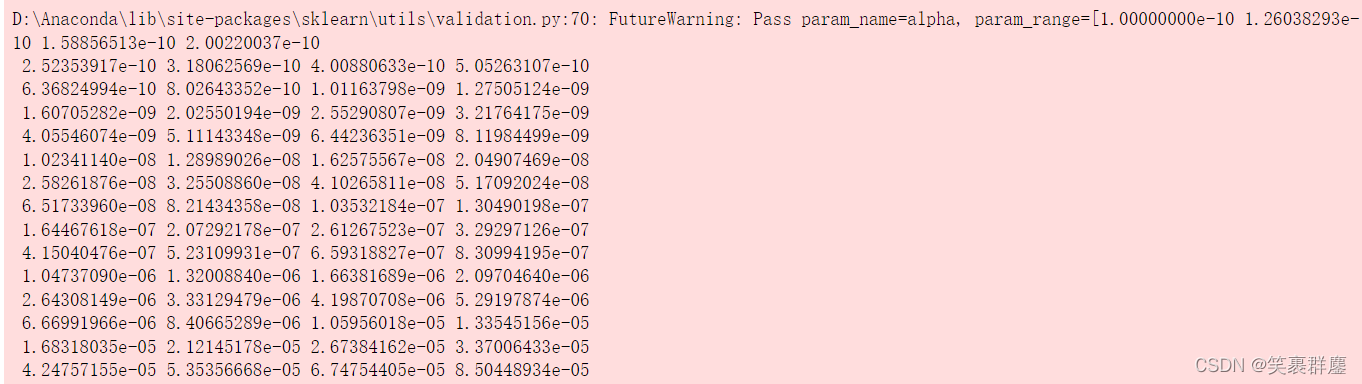（数据量过大，在此仅显示部分信息）

6、验证集的模型评分：

print(test_scores)


结果为：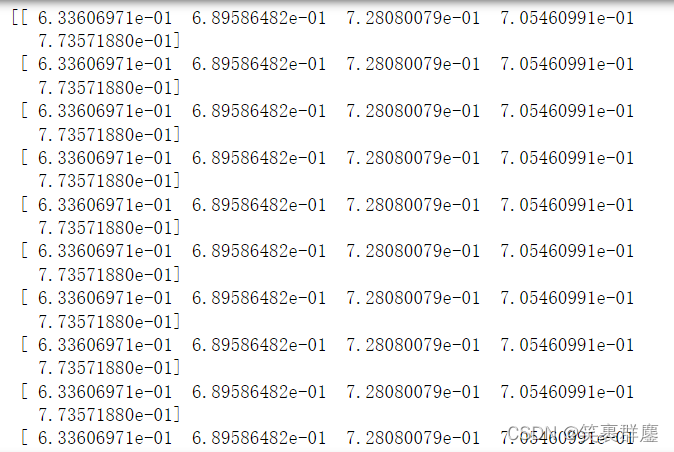（仅显示部分结果）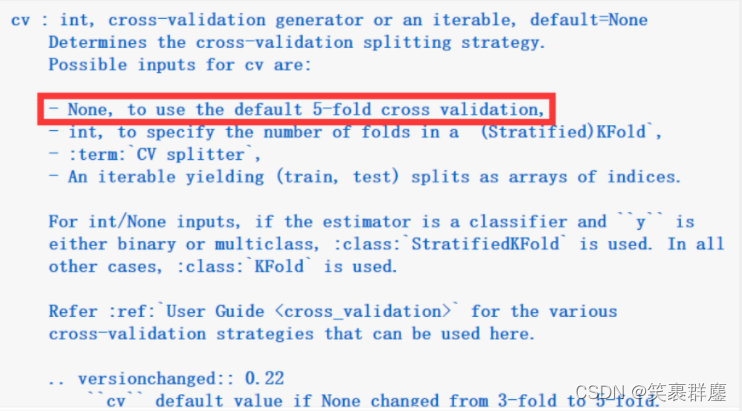7、绘制验证曲线：

import matplotlib.pyplot as plt
%matplotlib inline
plt.scatter(np.logspace(-10,10,200),np.mean(test_scores,axis = 1))


结果为：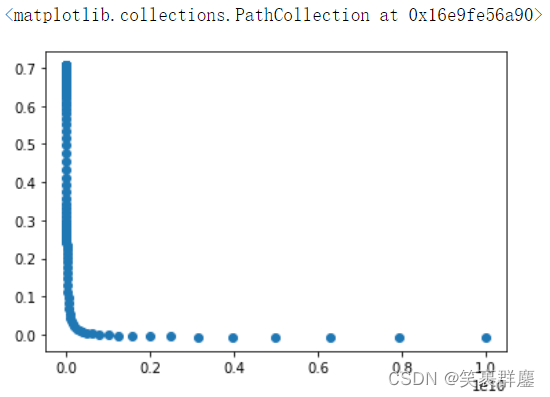## 学习曲线：

• 用于评估多大的样本量用于训练才能达到最佳效果

• 在初期，增加样本量会使得模型评分持续改善，但改善速度逐渐放缓

• 如果在增加训练集大小时，训练分值和验证分值都已经收敛到一个很低的
平稳值，则继续增加训练数据并不会改善模型效果

• 如果训练样本最大化时，训练分值仍然明显高于验证分值，说明模型存在
过拟合，此时增加训练样本很可能会改善模型的泛化能力

1、导入学习曲线模块：

from sklearn.model_selection import learning_curve


2、设置训练集大小：

size = np.linspace(0.1,1,10)


3、返回评价结果：

train_sizes,train_scores,test_scores = learning_curve(Ridge(),
X,y,
train_sizes = size,
cv = 10)


4、训练集大小：

print(train_sizes)


结果为：

[ 45  91 136 182 227 273 318 364 409 455]


5、训练集评分：

print(train_scores)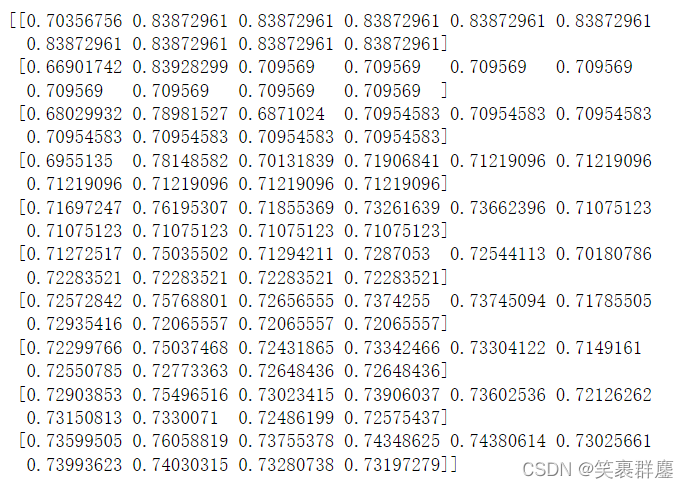6、验证集评分：

print(test_scores)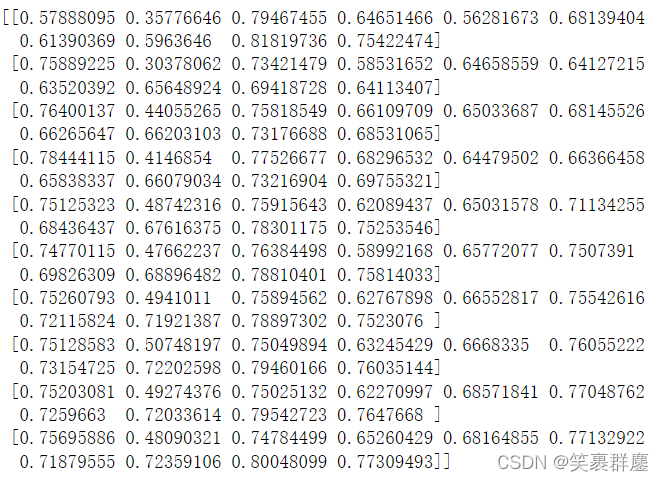7、绘制学习曲线：

plt.scatter(train_sizes,np.mean(train_scores,axis = 1))
plt.scatter(train_sizes,np.mean(test_scores,axis = 1))


结果为：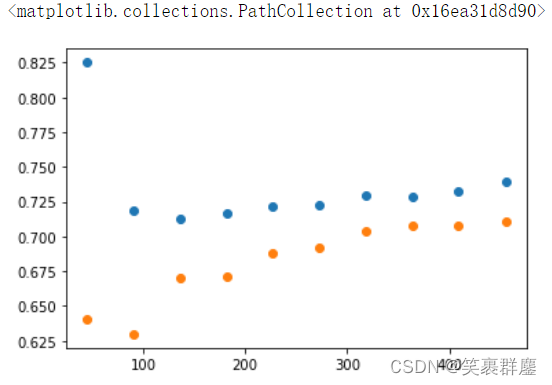8、更改训练集大小进行验证：

• 8.1更改训练集大小为100：
size = np.linspace(0.1,1,100)
plt.scatter(train_sizes,np.mean(train_scores,axis = 1),s = 2)
plt.scatter(train_sizes,np.mean(test_scores,axis = 1),s = 2)


结果为：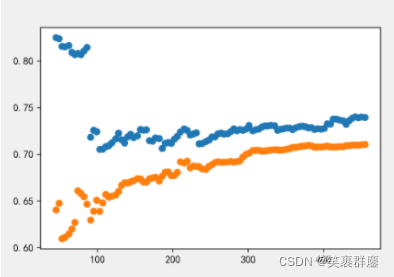• 8.2更改训练集大小为1000：
size = np.linspace(0.1,1,1000)
plt.scatter(train_sizes,np.mean(train_scores,axis = 1),s = 2)
plt.scatter(train_sizes,np.mean(test_scores,axis = 1),s = 2)


结果为：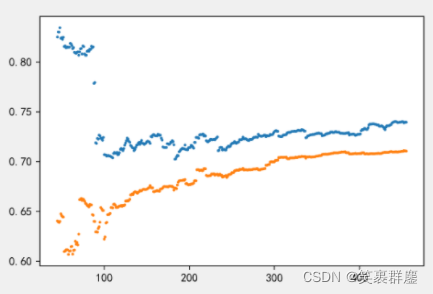展开全文python jupyter 机器学习 分类算法
• 验证曲线（ validation curve）是什么？如何绘制验证曲线（ validation curve）？验证曲线（ validation curve）详解及实践.pdf
• python sklearn 验证曲线 下载文件地址： 链接: https://pan.baidu.com/s/1VdjCSw9MfKZ1WAcaiDyi8w 提取码: wtck """ 验证曲线 对超参数 进行逐一验证 以验证取得最佳 超参数 """ import numpy as np import ...

## python sklearn 验证曲线

下载文件地址：
链接: https://pan.baidu.com/s/1VdjCSw9MfKZ1WAcaiDyi8w 提取码: wtck

### 验证参数max_depth

"""
验证曲线 对超参数 进行逐一验证  以验证取得最佳 超参数
"""
import numpy as np
import sklearn.preprocessing as sp
import sklearn.ensemble as se
import sklearn.model_selection as ms
import matplotlib.pyplot as mp

# 读取数据
print(lines.shape)

# 整理样本空间 并编码 （对列执行标签编码）
train_x, train_y = [], []
encoders = []  # 标签编码数组
for index, row in enumerate(lines.T):
encoder = sp.LabelEncoder()
if index < (len(lines.T) - 1):  # 训练样本 X
train_x.append(encoder.fit_transform(row))
else:
train_y = encoder.fit_transform(row)  # 训练样本结果 Y
encoders.append(encoder)

train_x = np.array(train_x).T  # 转置
train_y = np.array(train_y)

print(train_x.shape, train_y.shape)
print(train_x, train_y)

# 随机森林分类模型
model = se.RandomForestClassifier(
max_depth=9, n_estimators=140, random_state=7)

# 验证曲线  参数max_depth 分别使用 1-10 验证结果
# 最好效果为 9
train_scores, test_scores = ms.validation_curve(
model, train_x, train_y, "max_depth", np.arange(1, 11, 1), cv=5)
print(test_scores.mean(axis=1))

# 绘制验证曲线
mp.grid(linestyle=":")
mp.plot(np.arange(1, 11, 1),
test_scores.mean(axis=1),
"o-", color="dodgerblue", label="Validation Curve")
mp.legend()
mp.show()### 验证参数 n_estimators

"""
验证曲线 对超参数 进行逐一验证  以验证取得最佳 超参数
"""
import numpy as np
import sklearn.preprocessing as sp
import sklearn.ensemble as se
import sklearn.model_selection as ms
import matplotlib.pyplot as mp

# 读取数据
print(lines.shape)

# 整理样本空间 并编码 （对列执行标签编码）
train_x, train_y = [], []
encoders = []  # 标签编码数组
for index, row in enumerate(lines.T):
encoder = sp.LabelEncoder()
if index < (len(lines.T) - 1):  # 训练样本 X
train_x.append(encoder.fit_transform(row))
else:
train_y = encoder.fit_transform(row)  # 训练样本结果 Y
encoders.append(encoder)

train_x = np.array(train_x).T  # 转置
train_y = np.array(train_y)

print(train_x.shape, train_y.shape)
print(train_x, train_y)

# 随机森林分类模型
model = se.RandomForestClassifier(
max_depth=9, n_estimators=140, random_state=7)

# 验证曲线 n_estimators 分别使用 100-200 验证结果
train_scores, test_scores = ms.validation_curve(model, train_x, train_y, "n_estimators",
np.arange(100, 200, 10), cv=5)
print(test_scores.mean(axis=1))

# 绘制验证曲线
mp.grid(linestyle=":")
mp.plot(np.arange(100, 200, 10),
test_scores.mean(axis=1),
"o-", color="dodgerblue", label="Validation Curve")
mp.legend()
mp.show()展开全文• 最近在复盘优达学城的机器学习项目， 重新写了一下波斯顿放假预测的项目，除了模型的选择及网格搜索进行选择参数的相关知识外，还着重复习了关于学习曲线和复杂度曲线（验证曲线）  首先学习曲线和复杂度曲线...

最近在复盘优达学城的机器学习项目， 重新写了一下波斯顿放假预测的项目，除了模型的选择及网格搜索进行选择参数的相关知识外，还着重复习了关于学习曲线和复杂度曲线（验证曲线）

首先学习曲线和复杂度曲线（验证曲线）的区别，学习曲线是指在参数值确定的情况下，训练集和验证集的得分情况的对比，复杂度曲线（验证集曲线）是展示某个参数在取不同值时候，训练集与测试集得分情况的对比。

学习曲线的X轴是数据的数量，y轴是得分；复杂度曲线（验证曲线）的x轴是一个参数的值:比如max_depth（1,2,3,4,5,6,7,8,9,10），y轴是训练集和测试集在各个参数取值情况下的得分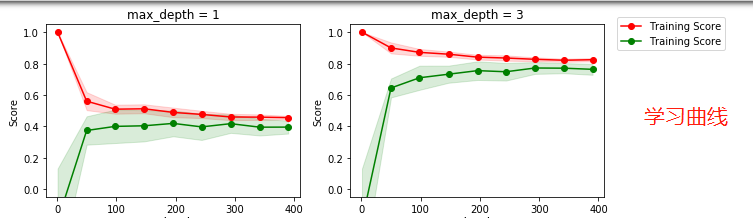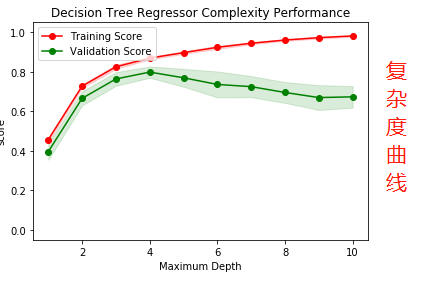上面两张图分别是学习曲线和复杂度曲线

下面来说一下学习曲线或者验证曲线的数据集合的划分

1 ShuffleSplit 划分

上面的两个图中就是用ShuffleSplit 方式对数据集合进行划分的，代码如下:

cv = ShuffleSplit(features.shape, n_iter = 10, test_size = 0.2, random_state = 0)
train_sizes = np.rint(np.linspace(1, data.shape*0.8 - 1, 9)).astype(int)

train_sizes, train_score, test_score = curves.learning_curve(
XGBRegressor(), X, y, cv=cv, train_sizes=train_sizes,scoring =score_fun
)

train_score_mean = np.mean(train_score,axis=1)
strain_score_std = np.std(train_score)
test_score_mean = np.mean(test_score,axis=1)
test_score_stu = np.stu(train_score,axis=1)

在学习曲线函数中，通过cv 参数来设置划分的数据集，有几种方式:

（1）如上边代码，cv 是按照某种方式划分好的数据集

（2）cv=10，这种cv 是数字格式，是直接采用n-fold 的交叉检验的方式进行，直接设置n的值即可

（3）自己手动划分

#数据集合中有490条数据 index 是从0-489
train_index = np.arange(0,391)
test_index = np.arange(391,489)

#构建cv 集合
test_cv = [[train_index,test_index]]

#学习曲线 cv参数传入test_cv
sizes,train_scores,test_scores = curves.learning_curve(regressor,features,prices,cv=test_cv,train_sizes=train_sizes)


下面看下学习曲线的画法，代码如下：

sizes,train_scores,test_scores = curves.learning_curve(regressor,features,prices,cv=cv,train_sizes=train_sizes)

train_std = np.std(train_scores, axis = 1)
train_mean = np.mean(train_scores, axis = 1)
test_std = np.std(test_scores, axis = 1)
test_mean = np.mean(test_scores, axis = 1)

depth = 4
ax.plot(sizes, train_mean, 'o-', color = 'r', label = 'Training Score')
ax.plot(sizes, test_mean, 'o-', color = 'g', label = 'Training Score')
ax.fill_between(sizes, train_mean - train_std,train_mean + train_std, alpha = 0.15, color = 'r')
ax.fill_between(sizes, test_mean - test_std, test_mean + test_std, alpha = 0.15, color = 'g')

ax.set_ylabel('Score')
ax.set_title('max_depth = %s'%(depth))
ax.set_ylim([-0.05, 1.05])


来看下这三种划分方式的学习曲线情况：

（1）shuffleSplit 划分：cv=ShuffleSplit（.......） cv=cv,对于这个数据集 很适合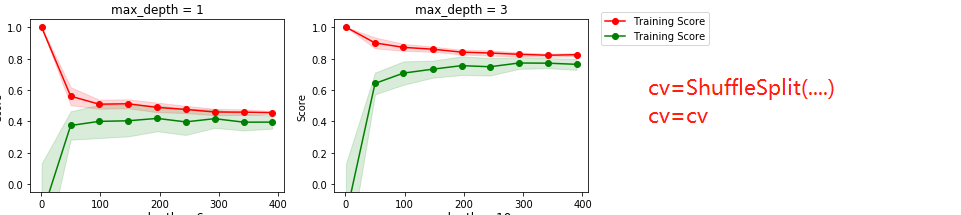(2) cv=10 交叉验证 n-fold 划分方式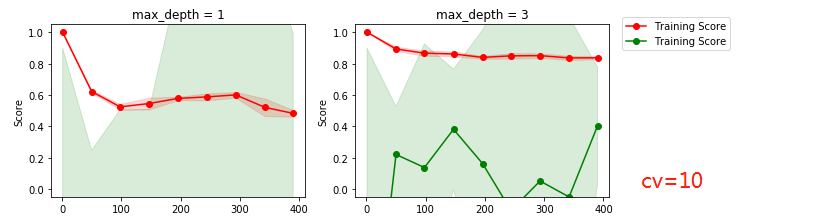可以看出学习曲线变化很大

（3） 手动划分 test_cv=[[train_index,test_index]] ,cv=test_cv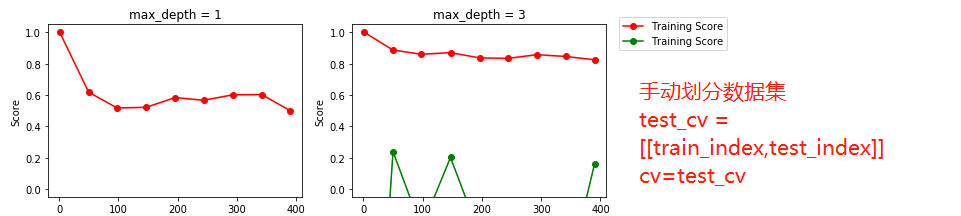由此可以看出，对于同一个数据集，划分的方式不同，其验证曲线会有很大差别 。

展开全文交叉检验
• 提高学习算法性能的简单但功能强大的判定工具：学习曲线，验证曲线 学习曲线 学习曲线能判定偏差和方差问题   高偏差与高方差： 以上模型应用于训练和交叉验证准确率很低，此模型未能很好打的拟合...Python 机器学习
• 机器学习好伙伴之scikit-learn的使用——验证曲线什么是验证曲线sklearn中验证曲线的实现应用示例 什么是验证曲线呢，其内容主要包含当超参数变化时，loss的变化情况。 什么是验证曲线 验证曲线主要反应的是当超...机器学习
• 在上一篇文章中，我们使用了交叉验证的方法来估计模型的平均性能，这篇文章中，将介绍通过学习曲线来判断模型的偏差和方差问题，通过验证曲线来判断模型参数对于模型的过拟合和欠拟合问题。一、学习曲线判断模型的...机器学习
• 验证曲线的作用 我们知道误差由偏差（bias）、方差（variance）和噪声（noise）责成。 偏差：模型对于不同的训练样本集，预测结果的平均误差。 方差：模型对于不同训练样本集的敏感程度 噪声：数据集本身的一项属性 ...
• scikit-learn中有非常多的曲线，对于调参非常有用处，我经常用到的曲线是学习曲线，验证曲线以及交叉验证曲线。 使用这些曲线的代码格式非常固定： 返回值 = 曲线名字（模型对象，X, Y, 交叉验证数量，评分标准......sklearn python 机器学习
• 验证曲线可以用来可视化分析，参数的优化调整对训练/测试数据集的性能影响。 FROM Sebastian Raschka, Vahid Mirjalili. Python机器学习第二版. 南京：东南大学出版社，2018. Section II: Code Bundle and ...机器学习 python
• 验证曲线 为了验证一个模型，我们需要分数函数，例如，分类器准确率。选择一个估计量的多个超参数的正确方式是网格搜索或类似的方法，这类方法选择在一个或多个验证集上具有最高分数的超参数。注意，如果我们根据...python
• 第四步： 画验证曲线  我们可以通过该随机森林中基分类器的个数，然后画出随机森林中验证集准确率与基分类器个数的关系 def get_validation_curve(X, y): classifer = RandomForestClassifier(max_depth=4...随机森林 Python 机器学习
• 1.模型的误差产生的机制 • 误差（Error）：模型预测结果与真实结果之间的差异 • 偏差（bias）：模型的训练误差叫做偏差 • 方差（Variance）：训练误差和测试误差的差异大小叫方差 1.1 欠拟合与过拟合 ...过拟合 欠拟合
• # -*- coding: utf-8 -*- ''' Created on 2018年1月18日 @author: Jason.F @summary: 判别过拟合和欠拟合 ...验证曲线validation Curve：评估参数和指标的关系 ''' import pandas as pd from sklearn.preprocessing
• 验证曲线（validation_curve）和学习曲线（sklearn.model_selection.learning_curve()）的区别是，验证曲线的横轴为某个超参数，如一些树形集成学习算法中的max_depth、min_sample_leaf等等。 从验证曲线上可以看到...
• 1、绘制验证曲线 在此图中，随着内核参数gamma的变化，显示了SVM的训练分数和验证分数。 对于非常低的gamma值，可以看到训练分数和验证分数都很低。这被称为欠配合。 gamma的中值是两个分数的高值，即分类器表现...
• 【利用sklearn库的 验证曲线 评估模型】   from sklearn.model_selection import validation_curve param_range = [0.001,0.01, 0.1, 1.0, 10.0, 100.0] train_scores, test_scores = validation_curve...
• 验证曲线的作用？ 验证曲线是什么？ 怎么解读？ 怎么画？ 验证曲线的作用？我们知道误差由偏差(bias)、方差(variance)和噪声(noise)组成。偏差：模型对于不同的训练样本集，预测结果的平均误差。 方差：模型对于...
• 在进行模型调参时：其他参数不变，绘制一个参数变化的训练曲线和验证曲线，选择训练效果和验证效果最佳时的参数。依次对每个参数进行绘制图形，调整得到相对优化的模型。 #继续使用随机森林建立模型 from ...
• test_scores.mean(axis=1), linewidth=2, c='dodgerblue') mp.show() 验证曲线 验证曲线: 模型性能(得分) = f(超参数) 什么是验证曲线   验证曲线是横轴为某个超参数的一系列值，由此来看不同参数设置下模型准确...决策树 python 机器学习 人工智能
• 函数与分段函数绘制函数曲线直接使用M文件使用函数 function分段函数定义和使用法1：分段函数的输入参数是单个的X值法2：定义分段函数的输入参数是向量X 绘制函数曲线 直接使用M文件 绘制曲线: y = xe(1-x) x为[0:...
• 目录1 交叉验证2 Python\rm{}PythonPython绘制交叉验证AUROC\rm{}AUROCAUROC3 Python\rm{}PythonPython绘制交叉验证AURR\rm{}AURRAURR4 参考文献 1 交叉验证    2 Python\rm{}PythonPython绘制交叉验证AUROC\rm{}...python AUROC
• 基于椭圆曲线上的双线性对技术，构造一种可验证秘密共享方案。该方案的信息率为 2/3，与 Pederson的方(Crypto91)及相关方案相比，本方案在相同的安全级别下有较高的信息率，从而提高了秘密共享协议的效率。同时，...
• 我们可以通过学习曲线来检测模型是欠拟合，过拟合还是刚刚好。
• 利用Caffe提供的脚本程序和Caffe训练日志画loss曲线与accuracy曲线。加强版，可以绘制对比曲线。辛辛苦苦修改作成的代码，大家还是给点儿资源分做鼓励吧，如果实在需要又没有资源分，请根据博客留下的联系方式联系我......